Type a math problemalgebra
trigonometry
statistics
calculus
matrices
variables
list
AC
log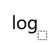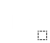ln
(
)
7
8
9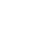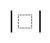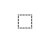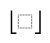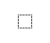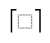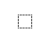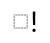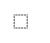τ
π
4
5
6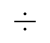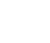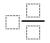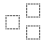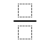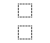%
θ
1
2
3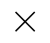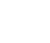<
>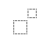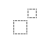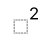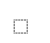x
i
0
.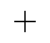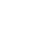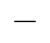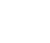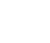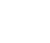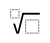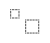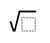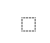y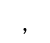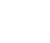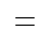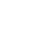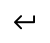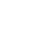Solve for nSteps for Solving Linear Equation
Variable cannot be equal to since division by zero is not defined. Multiply both sides of the equation by .
Use the distributive property to multiply by .
Subtract from both sides.
Combine and to get .
Subtract from both sides.
Subtract from to get .View solution steps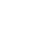Powered by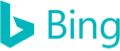3n+6=2\left(n-4\right)
Variable n cannot be equal to 4 since division by zero is not defined. Multiply both sides of the equation by n-4.
3n+6=2n-8
Use the distributive property to multiply 2 by n-4.
3n+6-2n=-8
Subtract 2n from both sides.
n+6=-8
Combine 3n and -2n to get n.
n=-8-6
Subtract 6 from both sides.
n=-14
Subtract 6 from -8 to get -14.